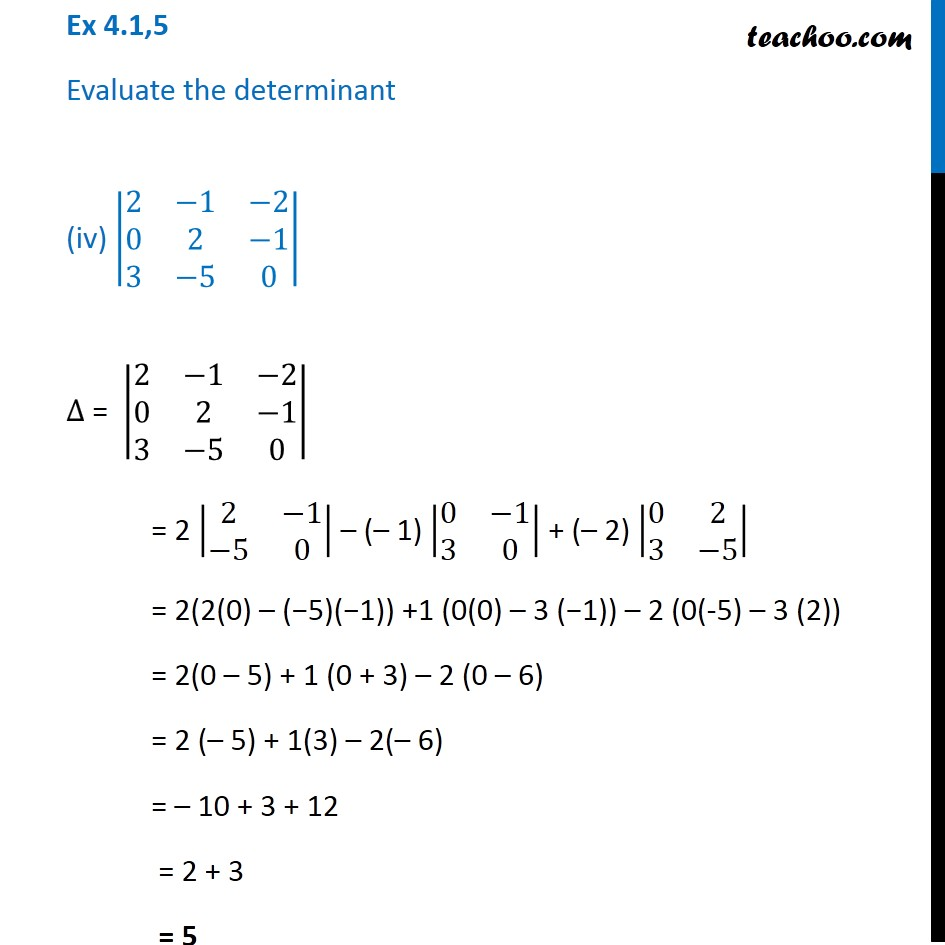1. Chapter 4 Class 12 Determinants (Term 1)
2. Serial order wise
3. Ex 4.1

Transcript

Ex 4.1,5 Evaluate the determinant (iv) |■8(2&−1&−2@0&2&−1@3&−5&0)| ∆ = |■8(2&−1&−2@0&2&−1@3&−5&0)| = 2 |■8(2&−1@−5&0)| – (– 1) |■8(0&−1@3&0)| + (– 2) |■8(0&2@3&−5)| = 2(2(0) – (−5)(−1)) +1 (0(0) – 3 (−1)) – 2 (0(-5) – 3 (2)) = 2(0 – 5) + 1 (0 + 3) – 2 (0 – 6) = 2 (– 5) + 1(3) – 2(– 6) = – 10 + 3 + 12 = 2 + 3 = 5